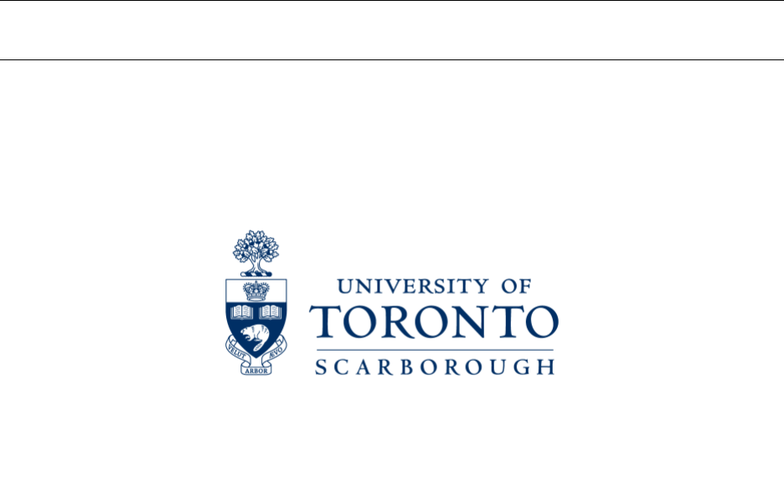Study Guides (390,000)
CA (150,000)
UTSC (10,000)
Final

# CSCA67H3 Lecture Notes - Lecture 9: Birthday Problem, Product Rule, Bayes EstimatorExam

Department
Computer Science
Course Code
CSCA67H3
Professor
Anna Bretscher
Study Guide
Final

This preview shows page 1. to view the full 5 pages of the document.Week 11, LEC02 - November 30th
Discrete Mathematics, Fall 2018
CSCA67 - Lecture Notes
Current Instructor: Dr. Richard Pancer
Instructors:
Dr. Anna Bretscher Dr. Richard Pancer
Email: bretscher@utsc.utoronto.ca pancer@utsc.utoronto.ca
Oﬃce: IC493 IC490
Oﬃce Hours: Monday 12:10 - 1:30 Monday 11:10 - 12:30
Wednesday 1:10 - 2:00 Friday 1:30 - 3:00
Friday 1:10 - 2:00 (will change after week 6)

Unlock to view full version

Only page 1 are available for preview. Some parts have been intentionally blurred.CSCA67 - Lecture Notes Richard Hong November 30th
Example. What is the probability of rolling two die whose sum is larger than 3?
Solution. We could add up P(4) + P(5) + P(6) + P(7) + P(8) + P(9) + P(10) + P(11) + P(12). What
is an easier way to compute this probability?
1 - P( sum = 2 ) P( sum = 3) = 1 1
36 2
36 =11
12
The Birthday Problem. What is the probability that in a group of n people at least two have the
same birthday?
Solution. Lets represent the days of the year by the integers 1, 2, . . . , 365 and use E to represent the
event that at least two people have the same birthday.
Q. What could we deﬁne our sample space S to be?
A. n tuples of integers from 1 to 365
Q.Lets assume all combinations or birthdays are equally likely. How many diﬀerent ways are there for
the n birthdays to fall?
A. |S|= 365n
Q. Is it easy to count the number of tuples with 2 or more values the same?
A. No. cannot be solved by directly scanning the sample space.
Q. How can we rephrase the problem to make it easier?
A. Compute the complementary probaility what is the event that everyone has a distinct(diﬀerent)
birthday?
Q. What if n > 365?
A. P(E) = 1, this is by Pigeon Hole Principle, if the birthdays are equally likely, by PHP ther will be
atleast two people with the same birthday.
so this counting problem is only for n365
Q. Suppose n 365. What is P(E)?
P(E) = 1 P(E)
A. There is only one way to count the number of ways to get distinct birthdays:
What is |E|?, |E|= 365 ·364 ·363 · · · · (365 n+ 1) = P(365, n)
Therefore P(E) = 1 - P(E) = 1 |E|
|S|= 1 P(365, n)
365n
This question is on the exam
Q. What value of n would you guess would result in a probability of at least 50% that two people have
the same birthday?
A. This equation is hard to solve....but if we simplify we get:
P(E) = 1 - P(E) = 1 P(365, n)
365n
P(E) 0.5
1P(365, n)
365n0.5. . . n 23
Page 1